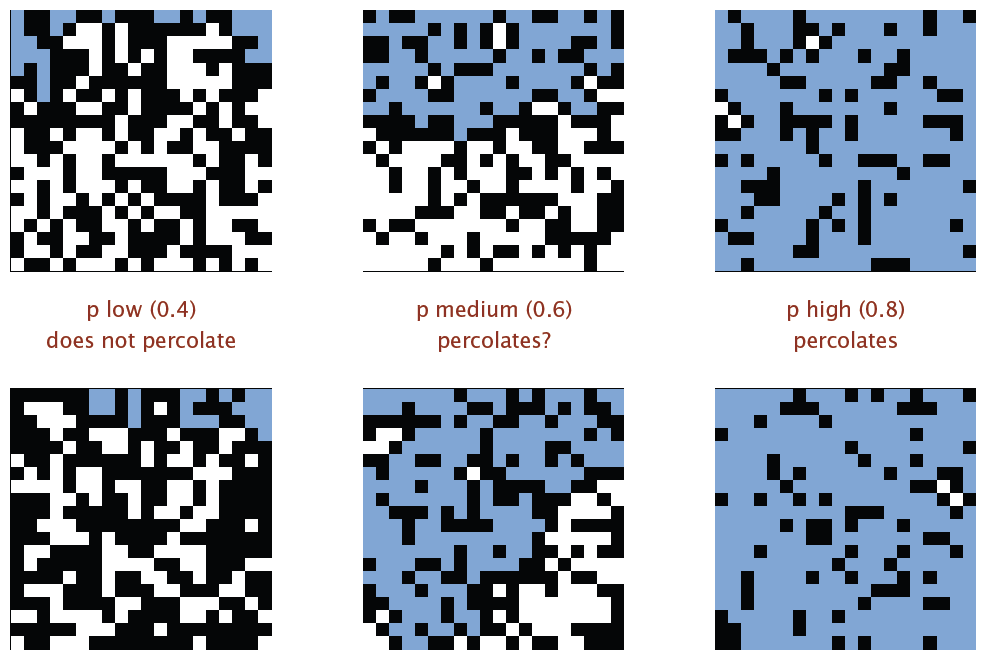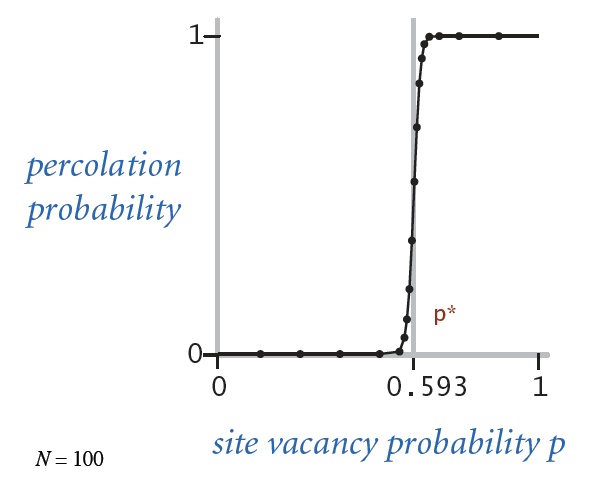# Using Go and Monte Carlo Method to obtain Percolation Threshold

This article is inspired by Slides in the Algorithms, Part I by Kevin Wayne, Robert Sedgewick. Image 1 and 2 are cropped from the original slide on page 51 and 52 of the 15UnionFind.PDF *

My knowledge is limited, my writing is most certainly flawed. Please don’t hesitate to leave me a feedback/suggestion/correction/rant

# Percolation and the threshold

In computer science, especially algorithm there is a topic that involves solving dynamic connectivity. Figuring out if a path/route is connected to other path/route is widely applicable in engineering the solutions to real-world problems.

For instance in many physical systems we have a model of N by N square grid, the blocks inside the grid can be either open or blocked. We call the system grid is percolated if and only if there is a path connecting top open sites with bottom open sites. Just like solving a labyrinth problem, on a massive grid it is very tiresome and ineffective (enumerate all possibility. Here checking whether two components are connected, a brute-force will cost $O(n^2)$ time) to obtain the percolating status. Therefore we need an efficient algorithm to solve this percolation problem. Determining whether 2 subset of the grid is connected can be modeled as connected components as in graph theory, which involves equivalence relation in the Set Theory:

On set X, an operation ~ is an equivalence relation iff a ~ a if a~b then b~a if a~b and b~c then a~c

Intuitively, subgraphs (components) are “equivalent” iff they have the same root. Because we don’t need to keep track of the shapes of the original subgraphs, we can model the solution to support a tree like data structure that is as flat as possible to guarantee the look-up speed, and some Connect (union) action to merge two subgraphs. In other words, we can choose Union Find algorithm to solve the percolation problem.

There is however a subsequent question regarding percolation that is more interesting: if all the grid blocks have a possibility p to be opened (blocked would be (1-p)), what is the likelihood that the whole system percolates? Imaging a social network with N by N user grid, each user has the possibility of p to be able to communicate with his/her neighbor, what are the odds that the first row users are linked with the bottom row users?We observed that above a certain p, the system most likely percolates. This critical threshold p is called Percolation Threshold. It appears to be a constant number, tied to the lattice structure.There is no direct solution to calculate the percolation threshold. But we sure can simulate the problem and if we do enough times of experiments, we will have some confidence that an approximation can be determined by the experiment results.

# Approach

Due to the fact that Simulations like Monte Carlo require iterating huge number of randomized experiments in order to deliver confident results, the number class we are talking here often start with millions. Running such simulations might be challenging if we have

1. Inefficient Experiments (software constraints)
2. Slow Computer (hardware constraints)

Point 1 might be overcome by using more efficient algorithms. However faster machines may not be available, especially since 2000 the trend of new computing engine is to go multi-core instead of higher frequency. This is why parallelism is often the way to go for such problems. I chose to use Go because on the one hand, it’s a fantastic system language which is fast, expressive and most familiar to C programmers; on the other hand, it has first class support for concurrency programming. Although the CSP paper by Hoare has been around for decades, there are very few of mainstream languages that adopt the concept.

# Concurrent Analysis

Each experiment in the simulation is independent to each other, this is a perfect entry-point where we can apply parallelism. Mainly we need to satisfy the condition

$$\begin{equation} P_{linear} (x) = P_{parallel} (x) \end{equation}$$
With
$$\begin{equation} P_{linear}(x) = \dfrac{\sum^n{p(x)}}{n} \end{equation}$$

where n is the number of all experiments, and $p(x)$ being the probability of experiment $x$ that yields a percolation.

And having denoted $w$ as the workload every worker has to perform and the $c$ is the count of all the works, the parallel version needs to deliver

$$\begin{equation} P_{parallel}(x) = \dfrac{\sum^c \sum^w{p(x)}}{n} \text{, with } w\cdot c = n \end{equation}$$

# The experiment

Ideally if we can have a grid constructed for us in a manner that it is instantly set up with randomized open sites, all we need to do is call the Find function on the grid (the $p(x)$ in previous section).

If I am to randomly fill up a completely blocked grid with open sites, it would take me maximal SIZE of the grid times to construct a percolation. Further, if I repeat the random construction again and again and record the steps that I took to produce a percolation in each and every experiment $\rightarrow$ when making a histogram of this recorded steps, I would have a plot that looks like a normal distribution which centers at the expected value. This expected value is exactly the wanted $p$ because the underlying pdf (probability density function) is what the plot represents.

To recap and modify our formula.

Let $s(x)$ be the steps used in $x$ experiment to produce a percolation; $n$ be the number of experiments; $g$ be the number of the blocks in the grid (size).

$$\begin{equation} P(x)=\dfrac{\sum^n{s(x)}}{n\cdot g} \end{equation}$$

Because $\dfrac{s(x)}{g} = p(x)$, we can parallel this approach on the top level too.

## High Level Algorithm

To randomly fill up open sites on a initially blocked square grid, is equivalent to obtaining a randomized permutation of numbers $0...N$-$1$, where $N$is the size of the grid. The rand package of the Go library can deliver that right out of the box.

On each site opening I need to connect the opened site to its neighbors, iff the neighbor is also opened.

Algorithm in Pseudo-code

Step := 0
LOOP number in Permutation
OBTAIN Neighbors of number
LOOP neighbor in Neighbors
IF neighbor is open
Connect / Union number with neighbor
Step++
IF Percolates / Find
RETURN Step
END IF
END IF
END LOOP
END LOOP


## Construction and Union Find

Now we need to construct the grid using cheap and smart data structures that supports our efficient union find algorithm. Let the size of the grid be $Size = N^2$, N is the length of its side. A weighted compressed Union-Find can achieve both union and find in $O(N+Mlg^*N)$time. * This Union-Find needs two arrays of size $N^2$, one (noted P) for presenting the parent relation / subgraph ownership of each element, the other (noted S) for the size of that component (used for weight comparison).

Additionally, in order to maintain states of the openness of the blocks, I need an boolean array of $N^2$. Finally, to ease the modeling of opening to top side edge and bottom side edge, we can add 2 virtual sites $N^2 + 1$ and $N^2 + 2$ to the original P array, the grid percolates iff

$$\begin{equation} N^2+1 \text{ and } N^2+2 \end{equation}$$
are connected.

# Code

Code contains 1 package called connectivity, which contains 2 components: unionfind and percolation. Both components are exported, you can use it on your own project. To run the simulation, please see next section.

Complete source code on Github

## Getting the code

go get github.com/nilbot/algo.go/connectivity



## Some Snippets

A Simulator interface:

type Simulator interface {
Simulate() int64
mark(n int)
Clear()
}


Data structure used in simulation

type PercolationSimulator struct {
Size    int
Marked  []bool
l       int
connect *WeightedCompressed
}


and its construction

func NewPercolationSimulator(N int) *PercolationSimulator {
result := &PercolationSimulator{
Size:    N * N,
Marked:  make([]bool, N*N),
l:       N,
connect: NewWeightedCompression(N*N + 2),
}
for i := 0; i != N; i++ {
result.connect.Union(i, result.Size)
}
for i := N * (N - 1); i != N*N; i++ {
result.connect.Union(i, result.Size+1)
}
return result
}


The 2 for loops are the trick to have 2 extra virtual sites connected to top and bottom edges, respectively.

To obtain a randomized permutation, calling (r *rand) Perm(int) from the math/rand package.

// return a slice of pseudo-random permutation of [0,n)
func getPermutation(n int) []int {
seed := time.Now().UnixNano() % 271828182833
r := rand.New(rand.NewSource(seed))
return r.Perm(n)
}


And marking open site, as outlined in pseudo-code in previous section:

// mark (paint) the block as white
func (p *PercolationSimulator) mark(n int) {
if p.Marked[n] {
return
}
p.Marked[n] = true
neighbors := getDirectNeighbours(n, p.l)
for _, adj := range neighbors {
}
}
}


For overview you can also visit the godoc

# Result and Benchmarks

## Run Test and Benchmark

cd \$GOPATH/github.com/nilbot/algo.go/connectivity
go test -bench Simulate


## Result

Benchmark

BenchmarkSimulate     100000         21375 ns/op


was achieved on a 2013 i7 8G MacbookAir. On the same machine running simulation with 10 million iterations yields

ran 1000000 simulations, got result 0.59377084

w